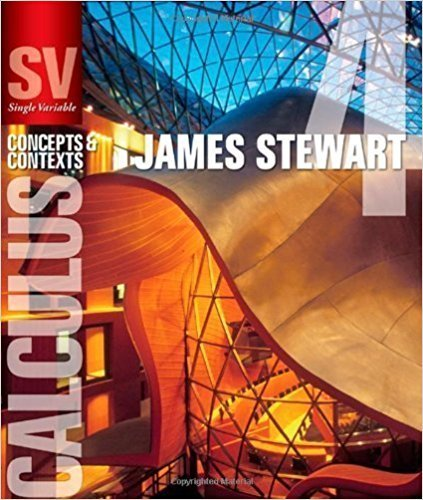×
×

# Solutions for Chapter 5.2: THE DEFINITE INTEGRAL## Full solutions for Single Variable Calculus: Concepts and Contexts (Stewart's Calculus Series) | 4th Edition

ISBN: 9780495559726Solutions for Chapter 5.2: THE DEFINITE INTEGRAL

Solutions for Chapter 5.2
4 5 0 257 Reviews
27
1
##### ISBN: 9780495559726

Single Variable Calculus: Concepts and Contexts (Stewart's Calculus Series) was written by and is associated to the ISBN: 9780495559726. Chapter 5.2: THE DEFINITE INTEGRAL includes 56 full step-by-step solutions. This expansive textbook survival guide covers the following chapters and their solutions. This textbook survival guide was created for the textbook: Single Variable Calculus: Concepts and Contexts (Stewart's Calculus Series), edition: 4. Since 56 problems in chapter 5.2: THE DEFINITE INTEGRAL have been answered, more than 20713 students have viewed full step-by-step solutions from this chapter.

Key Calculus Terms and definitions covered in this textbook
• Conversion factor

A ratio equal to 1, used for unit conversion

• Directed line segment

See Arrow.

• Ellipsoid of revolution

A surface generated by rotating an ellipse about its major axis

• General form (of a line)

Ax + By + C = 0, where A and B are not both zero.

• Graph of parametric equations

The set of all points in the coordinate plane corresponding to the ordered pairs determined by the parametric equations.

• Hyperboloid of revolution

A surface generated by rotating a hyperbola about its transverse axis, p. 607.

• Invertible linear system

A system of n linear equations in n variables whose coefficient matrix has a nonzero determinant.

• nth root

See Principal nth root

• nth root of unity

A complex number v such that vn = 1

• Order of an m x n matrix

The order of an m x n matrix is m x n.

• Parameter interval

See Parametric equations.

• Projection of u onto v

The vector projv u = au # vƒvƒb2v

• Pythagorean identities

sin2 u + cos2 u = 1, 1 + tan2 u = sec2 u, and 1 + cot2 u = csc2 u

• Quartic function

A degree 4 polynomial function.

• Quartile

The first quartile is the median of the lower half of a set of data, the second quartile is the median, and the third quartile is the median of the upper half of the data.

• Right triangle

A triangle with a 90° angle.

• Rigid transformation

A transformation that leaves the basic shape of a graph unchanged.

• Rose curve

A graph of a polar equation or r = a cos nu.

• Speed

The magnitude of the velocity vector, given by distance/time.